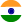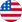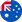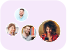24*7 support on WhatsAppChat NowProduct Edition:4th Edition
Author: Tomas Björk
Book Name: Arbitrage Theory in Continuous Time

# Arbitrage Theory in Continuous Time 4th Edition Solutions

0 out of 5.0
48 reviews24 Students
have requested for homework help from this book

The fourth edition of this widely used textbook on pricing and hedging of financial derivatives now also includes dynamic equilibrium theory and continues to combine sound mathematical principles with economic applications. Concentrating on the probabilistic theory of continuous time arbitrage pricing of financial derivatives, including stochastic optimal control theory and optimal stopping theory, Arbitrage Theory in Continuous Time is designed for graduate students in economics and mathematics, and combines the necessary mathematical background with a solid economic focus. It includes a solved example for every new technique presented, contains numerous exercises, and suggests further reading in each chapter. All concepts and ideas are discussed, not only from a mathematics point of view, but with lots of intuitive economic arguments. In the substantially extended fourth edition Tomas Björk has added completely new chapters on incomplete markets, treating such topics as the Esscher transform, the minimal martingale measure, f-divergences, optimal investment theory for incomplete markets, and good deal bounds. This edition includes an entirely new section presenting dynamic equilibrium theory, covering unit net supply endowments models and the Cox-Ingersoll-Ross equilibrium factor model. Providing two full treatments of arbitrage theory-the classical delta hedging approach and the modern martingale approach-this book is written so that these approaches can be studied independently of each other, thus providing the less mathematically-oriented reader with a self-contained introduction to arbitrage theory and equilibrium theory, while at the same time allowing the more advanced student to see the full theory in action. This textbook is a natural choice for graduate students and advanced undergraduates studying finance and an invaluable introduction to mathematical finance for mathematicians and professionals in the market.

5
24
4
10
3
9
2
10
1
0

0

## Students who viewed this book also checked out